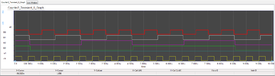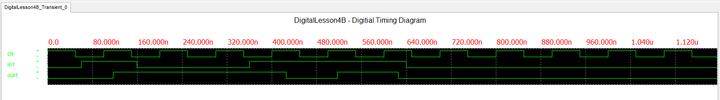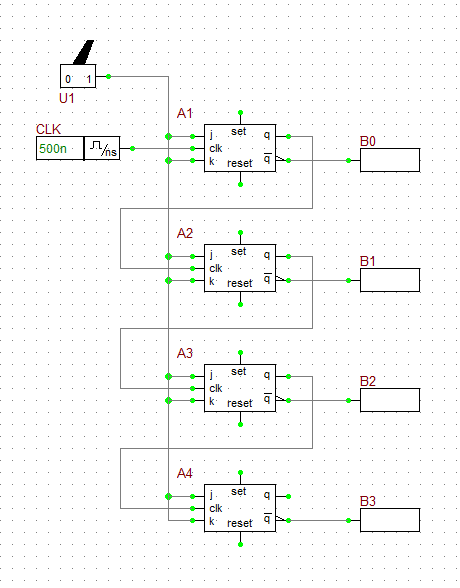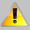# Basic Tutorial Lesson 11: Building a Binary Counter Using JK Flip-Flops

 Tutorial Project: Building a Binary Counter Using JK Flip-FlopsObjective: In this project, you will learn about JK flip-flops and will use them to build a 4-bit binary counter. Concepts/Features: JK Flip-Flop Toggle Flip-Flop Binary Counter Digital Timing Diagram Transient Test Time Step Ceiling Minimum Version Required: All versions

## What You Will Learn

In this tutorial you will first examine RF.Spice's JK flip-flop device and will then use four JK flip-flops to design a 4-bit binary counter. Besides using live timing diagrams, you will also learn how to run a transient analysis of your digital circuit.

## Testing a JK Flip-Flop

The following is a list of parts needed for this part of the tutorial lesson:

Part Name Part Type Part Value
In1 Digital Input 1-bit
In2 Digital Input 1-bit
Out1 Digital Output N/A
Out2 Digital Output N/A
CLK Digital Clock Period = 100ns, Pulse Width = 50ns
A1 JK-Type Flip-Flop Defaults

The JK Flip-Flop acts like a sequential (clocked) SR latch with its J and K inputs used for "Set" and "Reset" functions. The case of J = K = 0 represents the "Hold State". By contrast, the case of J = K = 1 is not illegal, but representing the "Toggle State". All the changes occur at the rising edge of the clock signal. Connect the JK Flip-Flop to the input and output devices as shown in the opposite figure.The JK-Type Flip-Flop device of RF.SPice A/D.

Set the time step to 20ns. Set both input values to zero initially. Run a live digital simulation and observe the timing diagram of the circuit. Step the simulation sequentially until t = 1200ns. Change the input values according to the table below:

Time In1 In2
0ns 0 0
60ns 1 0
160ns 0 1
360ns 1 1
640ns 0 0
800ns 0 1

The timing diagram is shown in the figure below. Note that the propagation delay times of the JK Flip-Flop device are tPLH = 16ns and tpHL = 25ns. The flip-flop is "set" at t = 60ns (J = 1 and K = 0). It takes until the clock's rising edge at t = 100ns for this change to take effect. The Q output of the flip-flop rises from 0 to 1 at t = 116ns and remains high. The flip-flop is then "reset" at t = 160ns (J = 0 and K = 1). It takes until the clock's rising edge at t = 200ns for this change to take effect. The Q output of the flip-flop falls from 1 to 0 at t = 225ns and then remains low. The first input rises to 1 again at t = 360ns. At this time, you will have J = K = 1, which represents the toggle state. At the clock's rising edge at t = 400ns, the toggle state takes effect. The output rises to 1 at t = 416ns and stays high. At the clock's next rising edge at t = 500ns, both J and K inputs are still high, therefore another toggle takes place. The output falls to 0 at t = 525ns and stays low. At the clock's next rising edge at t = 600ns, both J and K inputs are still high, and yet another toggle takes place. The output rises to 1 at t = 616ns and stays high. At t = 640ns, both inputs change to J = K = 0, representing the hold state. The output continues to maintain its high state even after the clock's next rising edge at t = 700ns. At t = 800ns, the flip-flop is reset (J = 0 and K = 1). It takes until the clock's next rising edge at t = 900ns for the reset to take effect. The output falls to 0 at t = 925ns and stays low until the end of the simulation.The timing diagram of the JK Flip-Flop circuit.

## Operating a JK Flip-Flop as a Toggle Flip-Flop

If you tie the two J and K inputs of a JK flip-flop together, it becomes equivalent to a T-Type (Toggle) Flip-Flop. A high input represents the "Toggle State", and a low input represents the "Hold State". In other words, when the input is high, the output toggles at the clock's rising edge. In the circuit of the previous part, delete the second digital input "In2". Then connect the K-pin of the flip-flop to its J-pin using the Wire Tool. The resulting circuit is shown in the figure below:The JK-Type flip-flop device configured as a Toggle flip-flop.

Activate the live timing diagram and step your digital circuit using the same scheme as in the previous part. The single input data are given in the table below:

Time In1
0ns 0
60ns 1
160ns 0
360ns 1
640ns 0
800ns 0

The resulting timing diagram is shown in the figure below. Note that when the input is one, the state of the output toggles on each rising edge of the clock. This happen at instants t = 100ns, 400ns, 500ns and 600ns. Also note the propagation delay that takes the output to toggle its binary state. The low-to-hight propagation delay of the flip-flop is 16ns, while its high-to-low delay is 25ns.The timing diagram of the JK Flip-Flop circuit configured as a Toggle flip-flop.

## Building a 4-Bit Binary Counter Using JK Flip-Flops

The following is a list of parts needed for this part of the tutorial lesson:

Part Name Part Type Part Value
U1 Toggle Switch N/A
B0 - B3 Digital Output N/A
CLK Digital Clock Period = 500ns, Pulse Width = 250ns
A1 - A4 JK-Type Flip-Flop Defaults
A5 - A7 Generic Inverter Gate Defaults

T flip-flops can be cascaded to build binary counters. In the last part of this tutorial lesson, you will use four JK flip-flops to build a 4-bit binary counter. Tie the J and K pins of all the four flip-flops together and connect them to the output of the Toggle Switch. Set the switch to the "1" (ON) state by clicking on the right side of its symbol. Set the Period of the clock CLK to 500ns and set its Pulse Width to 250ns. In this case, you can neglect the propagation delays compared to the clock period. Connect the digital clock device to the CLK pin of the first flip-flop, and connect output of the first flip-flop to the CLK pin of the second flip-flop. Cascade the four flip-flops in this way and connect all the outputs and inverters as shown in the figure below. The output of the first flip-flop provides the least significant bit B0, and the output of the fourth flip-flop provides the most significant bit B3.The 4-bit Binary Counter digital circuit.

## Running a Transient Test of the Binary Counter

Since digital simulations take place in the time domain, besides a live simulation, you can also run a transient test on them. Recall from the first few analog tutorial lessons that unlike live simulations, tests are preplanned simulations. In other words, in a test, the inputs are completely known and cannot change during the period of time when the simulation engine is doing its job. At the end of a test, you will see the final results. Contrast this to a live digital simulation, when the engine waits for you to make your next move. Then it responds to your action.

Next, open the Tests tab of the Toolbox, choose the Transient' test type and bring up the Transient Test Settings panel. Set the parameters for the transient test as follows:In the Transient Test of digital circuits, the input and output signals are automatically added to the graph signal list.
 Start Time 0 10u 20n 20n Defaults

Click the Run button to run the transient test. The following graph will appear at the bottom of the Workshop. This is indeed identical to the timing diagram you viewed in the previous step of the lesson. However, this digital graph is a regular RF.Spice A/D graph with full analysis and customization capability. For example, you can zoom in on the rising and falling edges of the pulses and examine the propagation delays.The transient response or finalized timing diagram of the 4-bit Binary Counter.

Since the input to all the flip-flops is high at all times, they are always in the toggle state. That means that the output of each flip-flop toggles its value at the rising edge of the clock of the flip-flop, which is itself the output of the previous stage. The first thing you observe from the figure is that each flip-flop functions as a frequency divider. Thus, the four flip-flops work as "Divide By 2", "Divide By 4", "Divide By 8" and "Divide By 16" frequency dividers, respectively, for the input clock CLK.

You have to wait until t = 500ns for all the toggle states to take effect. You can still see the cumulative propagation delays starting at t = 500ns. You will start measuring the outputs B0, B1, B2 and B3 at t = 750ns and at every clock cycle of 500ns thereafter. As you can see from the figure, at t = 750ns, B0 = B1 = B2 = B3 = 0. The 4-bit binary output is thus [B3][B2][B1][B0] = 0. After each rising edge of the input clock signal, the 4-bit binary output [B3][B2][B1][B0] is incremented by one. After 15 clock cycles, you get [B3][B2][B1][B0] = 1111 (equivalent to the decimal 15). After the sixteenth rising edge of the clock, the binary counter resets to zero again (e.g. at t = 8750ns). The results are given in the table below.

Time B3 B2 B1 B0
0ns 1 1 1 1
750ns 0 0 0 0
1250ns 0 0 0 1
1750ns 0 0 1 0
2250ns 0 0 1 1
2750ns 0 1 0 0
3250ns 0 1 0 1
3750ns 0 1 1 0
4250ns 0 1 1 1
4750ns 1 0 0 0
5250ns 1 0 0 1
5750ns 1 0 1 0
6250ns 1 0 1 1
6750ns 1 1 0 0
7250ns 1 1 0 1
7750ns 1 1 1 0
8250ns 1 1 1 1
8750ns 0 0 0 0